# Dirac Delta and Unit Heaviside Step Functions - Examples with Solutions

The Dirac delta function $\delta(t)$ and the Heavisisde unit step function $u(t)$ are presented along with examples and detailed solutions. These two functions are used in the mathematical modelling of various engineering systems. Some examples in modelling the responses of electric circuits to unit step voltages are included.



## Heaviside Unit Step Function $u(t)$

The unit Heaviside step function written as $u(t)$ (also called Heaviside function and written as $H(t)$ ) is defined as follows
$u(t) = \begin{cases} 0 & \text{for } t \lt 0 \\ 1 & \text{for } t \ge 0 \\ \end{cases}$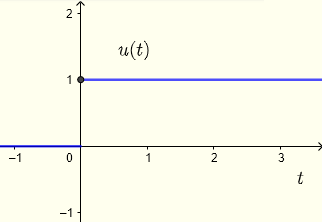Fig.1 - Graph of Unit Step Function
which therefore leads to
$u(t - t_0) = \begin{cases} 0, & \text{for } t \lt t_0 \\ 1, & \text{for } t \ge t_0 \\ \end{cases}$
One of the main uses of the step function is to model a switch for example.
Suppose we need to apply a voltage $v(t)$ to a circuit at the time $t = t_0$, the voltage as a function of time may be represented by $v(t) u(t-t_0)$ so that
$v(t) u(t-t_0) \begin{cases} v(t) &\mbox{if } t \ge t_0 \\ 0 & \mbox{if } t \lt t_0 \end{cases}$
An example, the graph of $t^2 u(t-1)$ is shown below.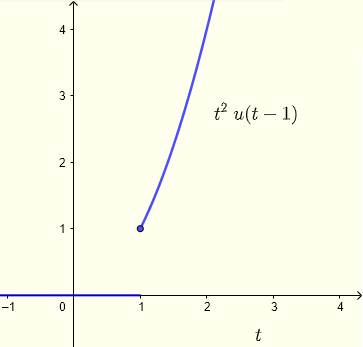Fig.2 - Unit Step Function Used to Model a Switch Additions and subtractions may of unit step functions may be used to model pulses; an example is shown below.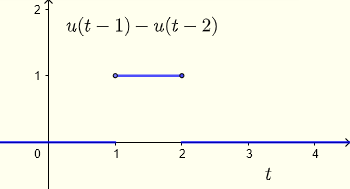Fig.3 - Unit Step Function Used to Model a Pulse

## Dirac Delta Function $\delta(t)$

The Dirac delta function is defined by the integral
$\displaystyle \int_{-\infty}^{t} \delta (\tau - t_0) d\tau = u(t - t_0)$
Although the unit step function $u(t - t_0)$ is discontinuous at $t = t_0$, we may define the derivative of the unit step function by the Dirac delta function as follows
$\dfrac{d u(t - t_0)}{dt} = \delta (t - t_0)$
which may take a "very large" value at $t = t_0$ and hence the Dirac delta function may also be viewed as
$\delta(t - t_0) = \begin{cases} \infty & \text{for } t = t_0 \\ 0 & \text{for } t \ne t_0 \\ \end{cases}$
The Dirac delta function defines the derivative at a finite discontinuity; an example is shown below.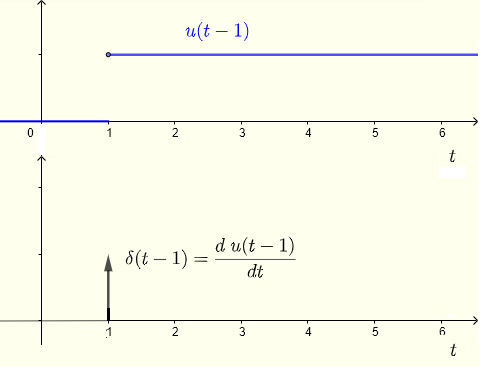Fig.4 - Graphical Relationship Between Dirac delta function and Unit Step Function The Dirac delta function has the following properties:
$\delta(t - t_0)$ is equal to zero everywhere except at $t = t_0$ hence the properties 1, 2 and 3.
1. $\displaystyle \int_{a}^{b} f(t) \delta (t - t_0) dt = f(t_0)$ if $a \lt t_0 \lt b$     ( or $t_0$ is inside the interval of integration ).

2. $\displaystyle \int_{a}^{b} f(t) \delta (t - t_0) dt = 0$ if $t_0 \gt b$ or $t_0 \lt a$     ( or $t_0$ is outside the interval of integration ).

3. $\displaystyle \int_{-\infty}^{\infty} \delta (t) dt = 1$

4. $\delta (t - t_0) = \delta (t_0 - t)$ because $\delta(t)$ is an even function

5. $f(t) \delta (t - t_0) = f(t_0) \delta (t - t_0)$

6. $\displaystyle \delta(t) = \dfrac{1}{2\pi} \int_{\infty}^{\infty} e^{ipt} dp$

7. $\delta( k t) = \dfrac{1}{|k|} \delta(t)$ for $k \ne 0$

## Examples with Solutions

Example 1
Evaluate the integrals:
a) $\displaystyle \int_{-\infty}^{\infty} \delta(t) e^{t^2+1} dt$      b) $\displaystyle \int_{-\infty}^{\infty} \delta(t-4) e^{2 \cos(0.5 \pi t)} dt$      c) $\displaystyle \int_{0^{-}}^{\infty} \delta(t) (t^2 + e^{-t}) dt$      d) $\displaystyle \int_{0}^{\infty} \delta(t + 3) e^{3t} dt$      e) $\displaystyle \int_{0^{+}}^{\infty} \delta(t) \sin(3t) dt$
Solution to Example 1

a) $\displaystyle \int_{-\infty}^{\infty} \delta(t) e^{t^2+1} dt = \int_{-\infty}^{\infty} \delta(t - 0) e^{t^2+1} dt = e^{0^2+1} = e^1 = e$      applying property 1 above since $-\infty \lt 0 \lt \infty$

b) $\displaystyle \int_{-\infty}^{\infty} \delta(t-4) e^{2 \cos(0.5 \pi t)} dt = e^{\cos(0.5 \pi (4) )} = e^{ 2 \cos (2\pi) } = e^2$      applying property 1 above since $-\infty \lt 4 \lt \infty$

c) $\displaystyle \int_{0^{-}}^{\infty} \delta(t) (t^2 + e^{-t}) dt = \int_{0^{-}}^{\infty} \delta(t-0) (t^2 + e^{-t}) dt = 0^2 + e^{0} = 1$      applying property 1 above since $0^- \lt 0 \lt \infty$

d) $\displaystyle \int_{0}^{\infty} \delta(t + 3) e^{3t} dt = \int_{0}^{\infty} \delta(t - (-3) ) e^{3t} dt = 0$      applying property 2 above since $- 3 \lt 0$ or $-3$ is outside the interval of integration.

e) $\displaystyle \int_{0^{+}}^{\infty} \delta(t) \sin(3t) dt = \int_{0^{+}}^{\infty} \delta(t - 0) \sin(3t) dt = 0$      applying property 2 above since $0 \lt 0^+$ or $0$ is outside the interval of integration.

Example 2
Evaluate the derivatives to:
a) $f(t) = u(t) - u(t-1)$      b) $f(t) = 2 u(t) - 3 u(t-2)$
Solution to Example 2
a) $f'(t) = \delta(t) - \delta(t-1)$
b) $f'(t) = 2 \delta(t) - 3 \delta(t-2)$

Example 3
Use the step function $u(t)$ to write equations to the graphs shown below and their derivatives.
a)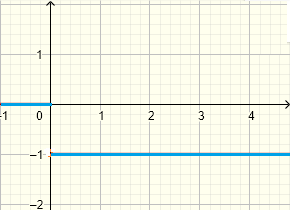b)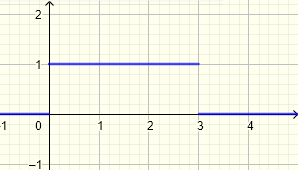c)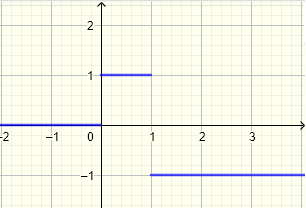d)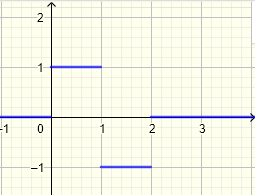Solution to Example 3
a) $f(t) = - u(t)$ , $f'(t) = - \delta(t)$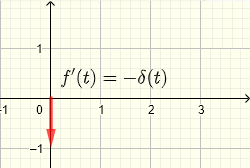b) $f(t) = u(t) - u(t-3)$ , $f'(t) = \delta(t) - \delta(t-3)$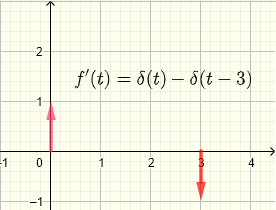c) $f(t) = u(t) - 2 u(t-1)$ , $f'(t) = \delta(t) - 2 \delta(t-1)$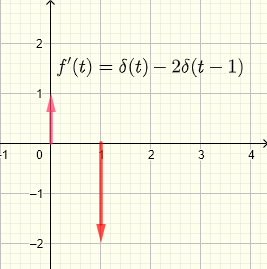d) $f(t) = u(t) - 2 u(t-1) + u(t-2)$ , $f'(t) = \delta(t) - 2 \delta(t-1) + \delta (t-2)$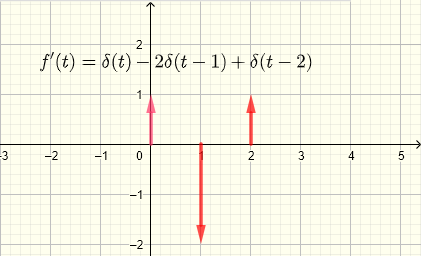## More References and Links

Heaviside step function